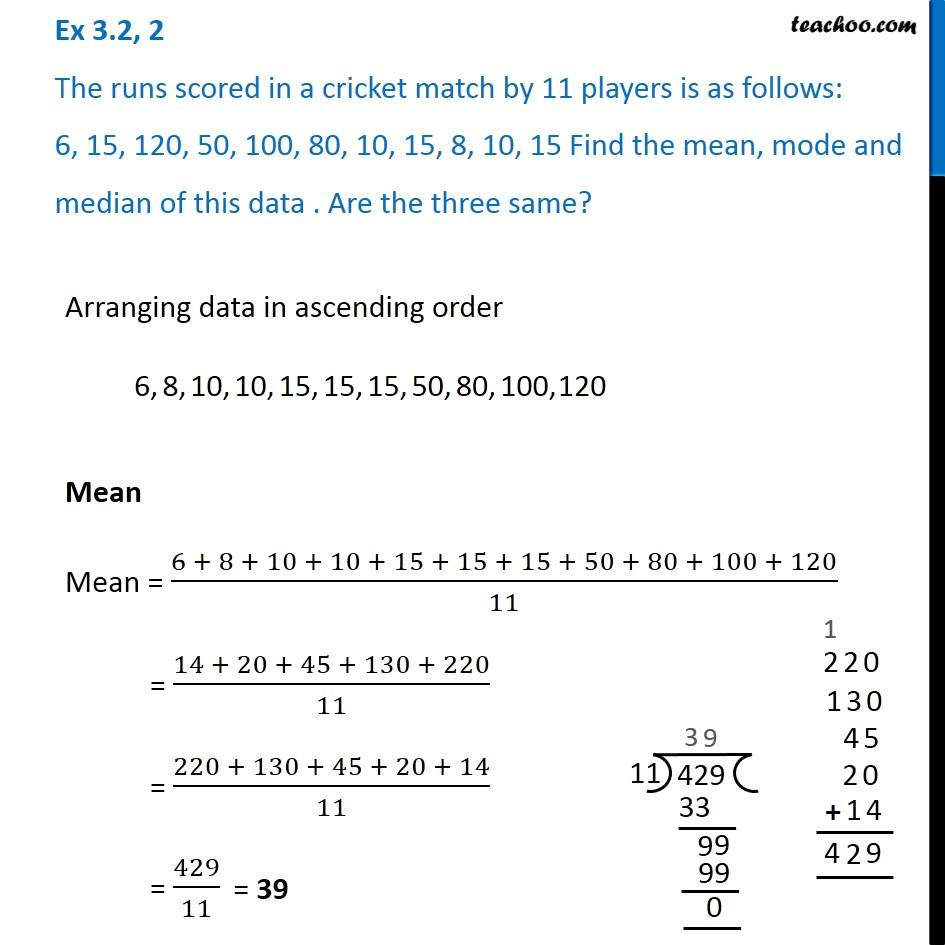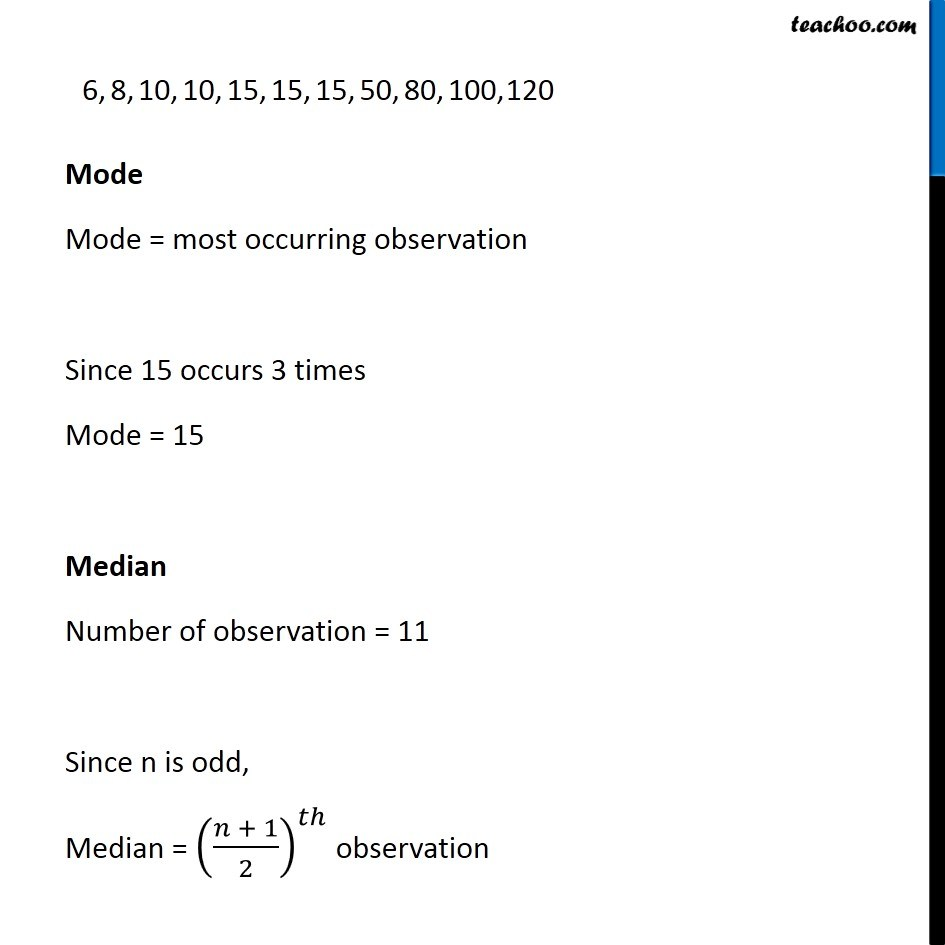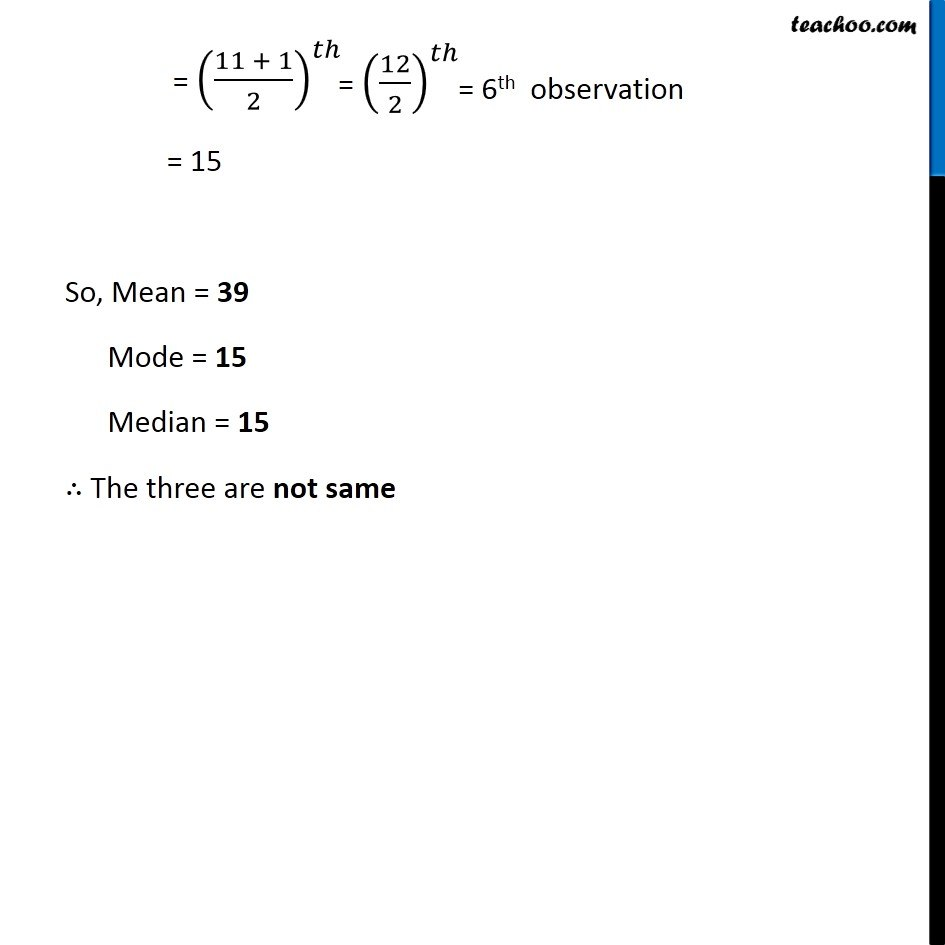Ex 3.2

Chapter 3 Class 7 Data Handling
Serial order wiseLearn in your speed, with individual attention - Teachoo Maths 1-on-1 Class

### Transcript

Ex 3.2, 2 The runs scored in a cricket match by 11 players is as follows: 6, 15, 120, 50, 100, 80, 10, 15, 8, 10, 15 Find the mean, mode and median of this data . Are the three same? Arranging data in ascending order Mean Mean = (6 + 8 + 10 + 10 + 15 + 15 + 15 + 50 + 80 + 100 + 120)/11 = (14 + 20 + 45 + 130 + 220)/11 = (220 + 130 + 45 + 20 + 14)/11 = 429/11 Mode Mode = most occurring observation Since 15 occurs 3 times Mode = 15 Median Number of observation = 11 Since n is odd, Median = ((𝑛 + 1)/2)^𝑡ℎ observation = ((11 + 1)/2)^𝑡ℎ = 15 So, Mean = 39 Mode = 15 Median = 15 ∴ The three are not same